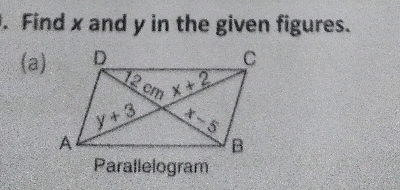"
">

# Find $x$ and $y$ in the given figures."

Given: $ABCD$ is a parallelogram.

To do: To find $x$ and $y$.

Solution:

$\because ABCD$ is a parallelogram, then diagonals $AC$ and $BD$ are equal. And form two congruent triangles.

$\Rightarrow y+3=x+2$

$\Rightarrow x-y=1\ ........\ ( i)$

And $x-5=12$

$\Rightarrow x==12+5=17$, On substituting $x=17$ in $( i)$,

$17-y=1$

$\Rightarrow y=17-1$

$\Rightarrow y=16$

Thus, $x=17$ and $y=16$.

Updated on: 10-Oct-2022

20 Views